Скачать презентацию Chapter 5 Practice Quiz Price Elasticity of Demand

da5a029a53fb93d636f283d5398866fe.ppt

• Количество слайдов: 17Chapter 5 Practice Quiz Price Elasticity of Demand Supply 1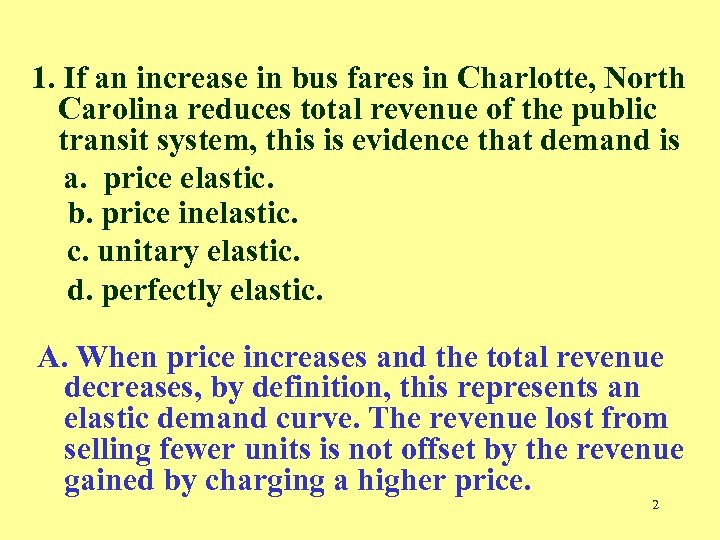1. If an increase in bus fares in Charlotte, North Carolina reduces total revenue of the public transit system, this is evidence that demand is a. price elastic. b. price inelastic. c. unitary elastic. d. perfectly elastic. A. When price increases and the total revenue decreases, by definition, this represents an elastic demand curve. The revenue lost from selling fewer units is not offset by the revenue gained by charging a higher price. 2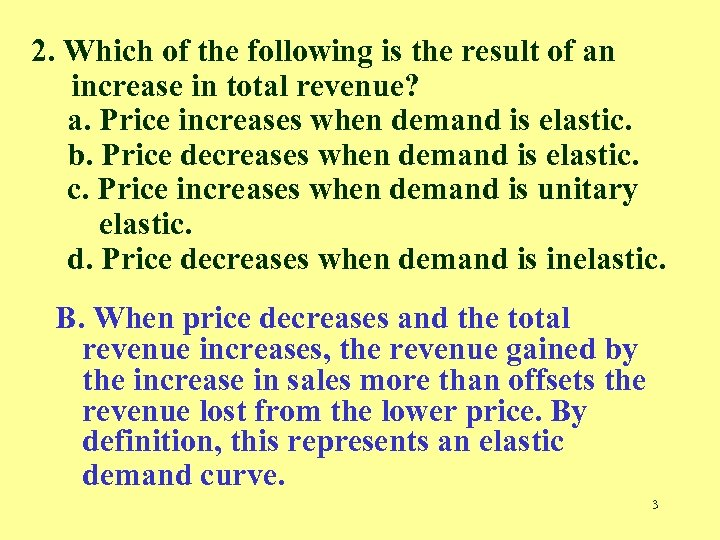2. Which of the following is the result of an increase in total revenue? a. Price increases when demand is elastic. b. Price decreases when demand is elastic. c. Price increases when demand is unitary elastic. d. Price decreases when demand is inelastic. B. When price decreases and the total revenue increases, the revenue gained by the increase in sales more than offsets the revenue lost from the lower price. By definition, this represents an elastic demand curve. 3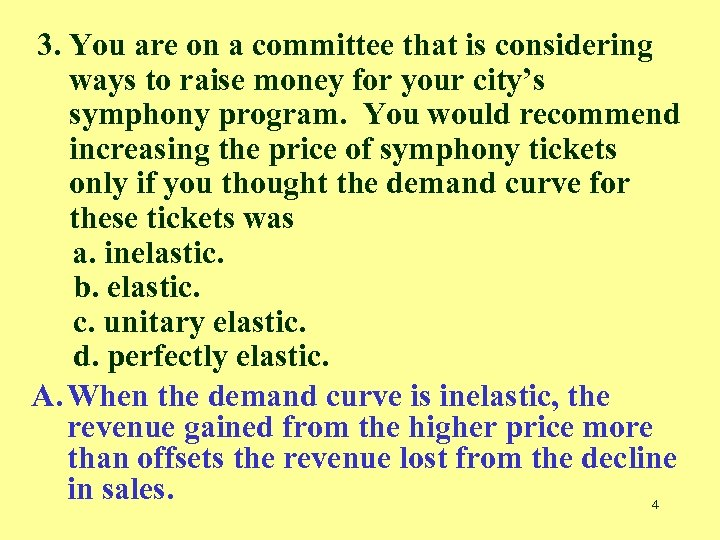3. You are on a committee that is considering ways to raise money for your city’s symphony program. You would recommend increasing the price of symphony tickets only if you thought the demand curve for these tickets was a. inelastic. b. elastic. c. unitary elastic. d. perfectly elastic. A. When the demand curve is inelastic, the revenue gained from the higher price more than offsets the revenue lost from the decline in sales. 4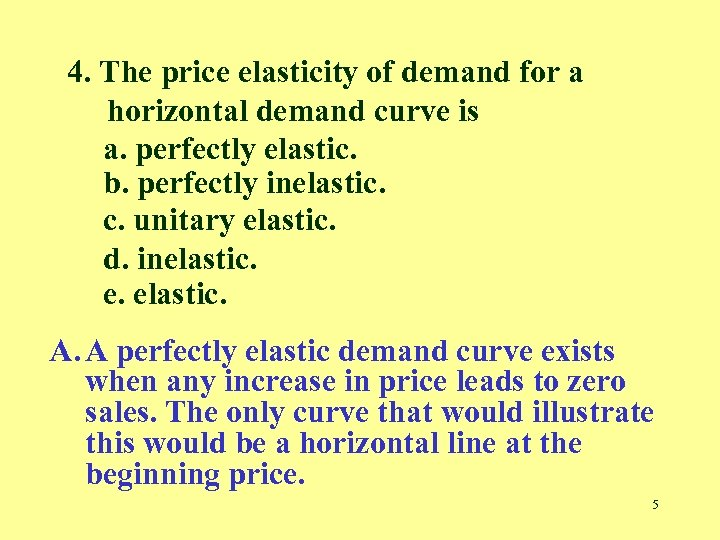4. The price elasticity of demand for a horizontal demand curve is a. perfectly elastic. b. perfectly inelastic. c. unitary elastic. d. inelastic. e. elastic. A. A perfectly elastic demand curve exists when any increase in price leads to zero sales. The only curve that would illustrate this would be a horizontal line at the beginning price. 5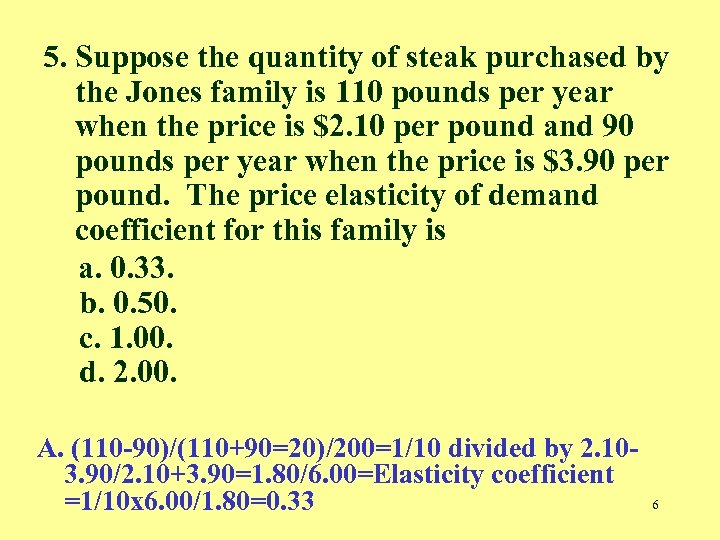5. Suppose the quantity of steak purchased by the Jones family is 110 pounds per year when the price is \$2. 10 per pound and 90 pounds per year when the price is \$3. 90 per pound. The price elasticity of demand coefficient for this family is a. 0. 33. b. 0. 50. c. 1. 00. d. 2. 00. A. (110 -90)/(110+90=20)/200=1/10 divided by 2. 103. 90/2. 10+3. 90=1. 80/6. 00=Elasticity coefficient =1/10 x 6. 00/1. 80=0. 33 6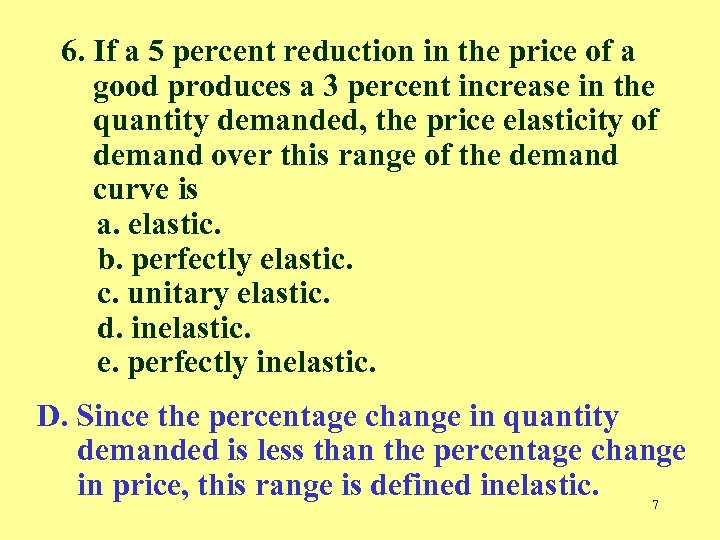6. If a 5 percent reduction in the price of a good produces a 3 percent increase in the quantity demanded, the price elasticity of demand over this range of the demand curve is a. elastic. b. perfectly elastic. c. unitary elastic. d. inelastic. e. perfectly inelastic. D. Since the percentage change in quantity demanded is less than the percentage change in price, this range is defined inelastic. 7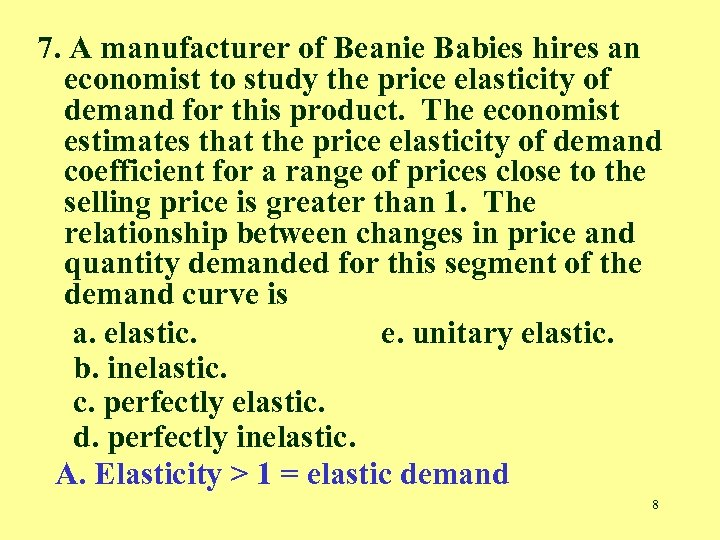7. A manufacturer of Beanie Babies hires an economist to study the price elasticity of demand for this product. The economist estimates that the price elasticity of demand coefficient for a range of prices close to the selling price is greater than 1. The relationship between changes in price and quantity demanded for this segment of the demand curve is a. elastic. e. unitary elastic. b. inelastic. c. perfectly elastic. d. perfectly inelastic. A. Elasticity > 1 = elastic demand 8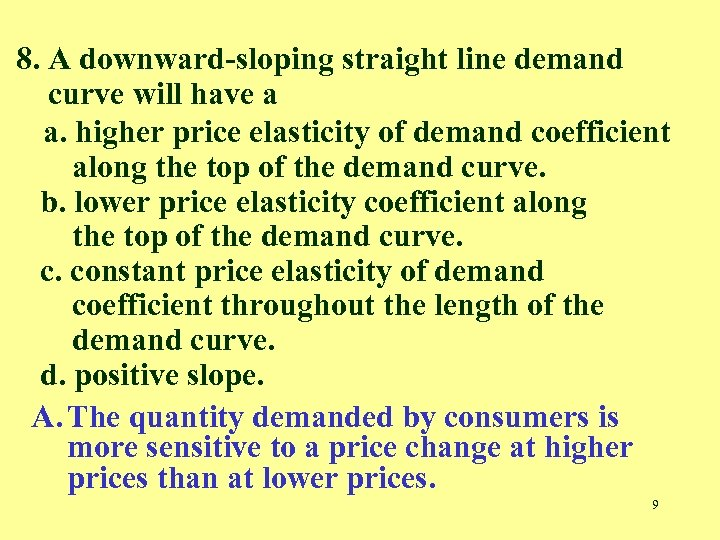8. A downward-sloping straight line demand curve will have a a. higher price elasticity of demand coefficient along the top of the demand curve. b. lower price elasticity coefficient along the top of the demand curve. c. constant price elasticity of demand coefficient throughout the length of the demand curve. d. positive slope. A. The quantity demanded by consumers is more sensitive to a price change at higher prices than at lower prices. 9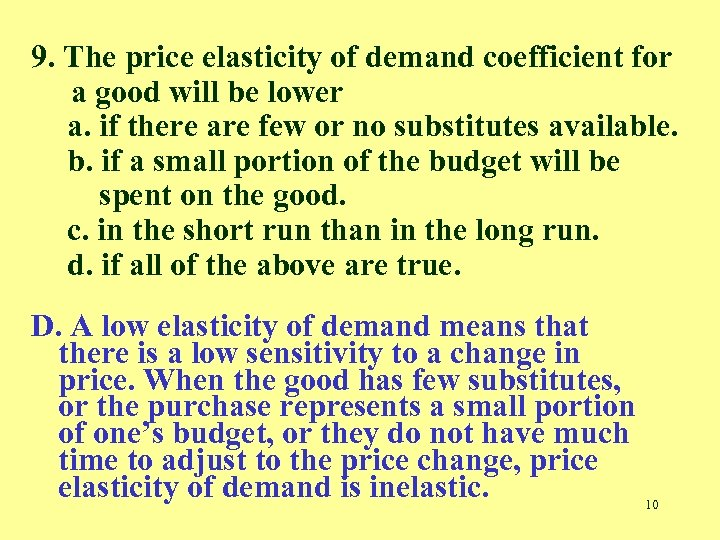9. The price elasticity of demand coefficient for a good will be lower a. if there are few or no substitutes available. b. if a small portion of the budget will be spent on the good. c. in the short run than in the long run. d. if all of the above are true. D. A low elasticity of demand means that there is a low sensitivity to a change in price. When the good has few substitutes, or the purchase represents a small portion of one’s budget, or they do not have much time to adjust to the price change, price elasticity of demand is inelastic. 10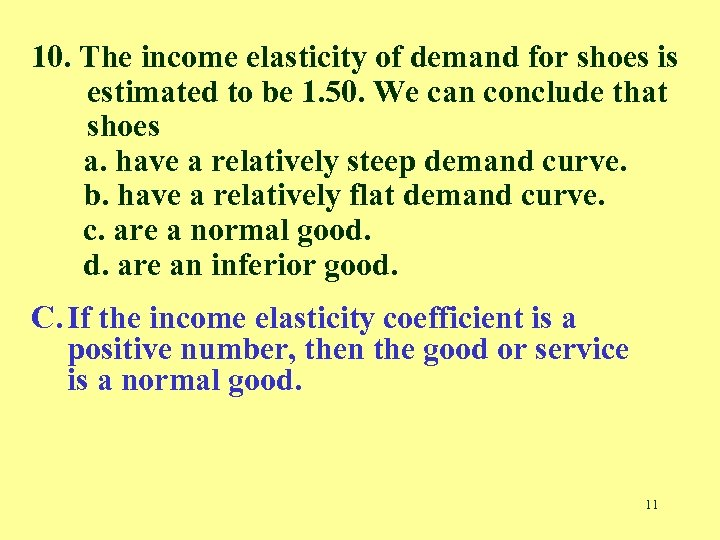10. The income elasticity of demand for shoes is estimated to be 1. 50. We can conclude that shoes a. have a relatively steep demand curve. b. have a relatively flat demand curve. c. are a normal good. d. are an inferior good. C. If the income elasticity coefficient is a positive number, then the good or service is a normal good. 11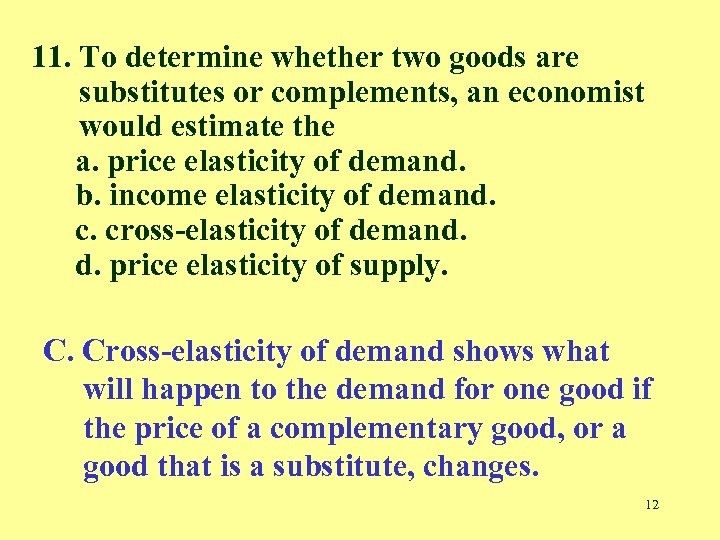11. To determine whether two goods are substitutes or complements, an economist would estimate the a. price elasticity of demand. b. income elasticity of demand. c. cross-elasticity of demand. d. price elasticity of supply. C. Cross-elasticity of demand shows what will happen to the demand for one good if the price of a complementary good, or a good that is a substitute, changes. 12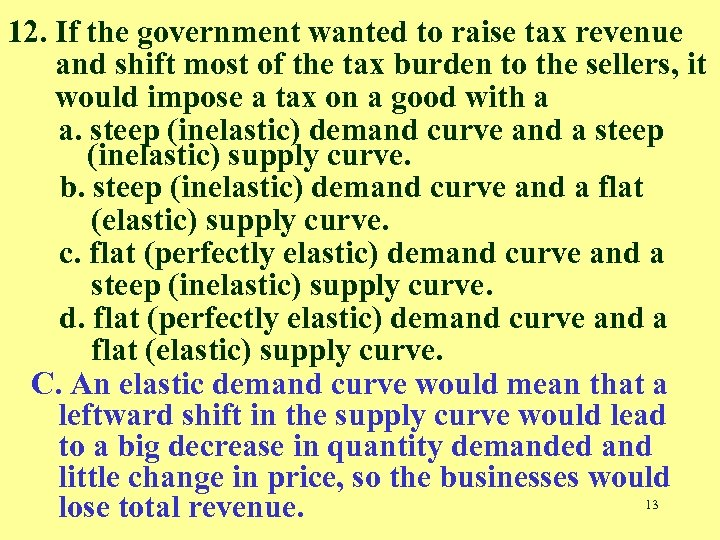12. If the government wanted to raise tax revenue and shift most of the tax burden to the sellers, it would impose a tax on a good with a a. steep (inelastic) demand curve and a steep (inelastic) supply curve. b. steep (inelastic) demand curve and a flat (elastic) supply curve. c. flat (perfectly elastic) demand curve and a steep (inelastic) supply curve. d. flat (perfectly elastic) demand curve and a flat (elastic) supply curve. C. An elastic demand curve would mean that a leftward shift in the supply curve would lead to a big decrease in quantity demanded and little change in price, so the businesses would 13 lose total revenue.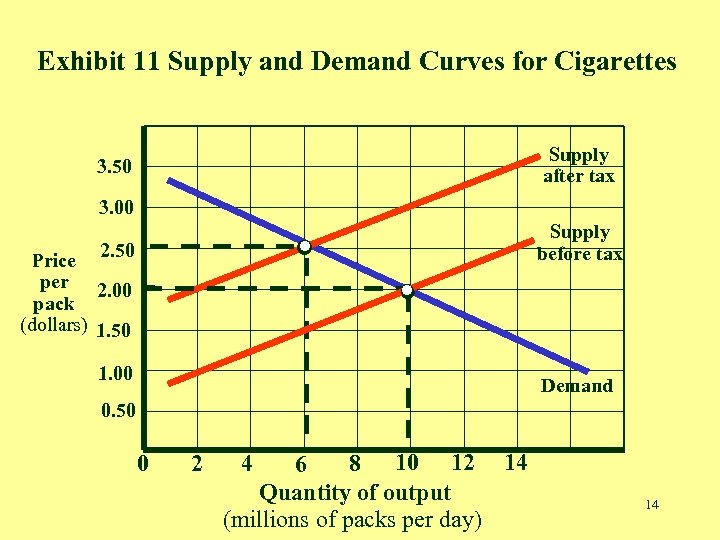Exhibit 11 Supply and Demand Curves for Cigarettes . . . 3. 50 . 3. 00 2. 50 Price per 2. 00 pack (dollars) 1. 50 1. 00 Supply after tax Supply before tax Demand 0. 50 0 2 8 10 12 14 6 Quantity of output (millions of packs per day) 4 14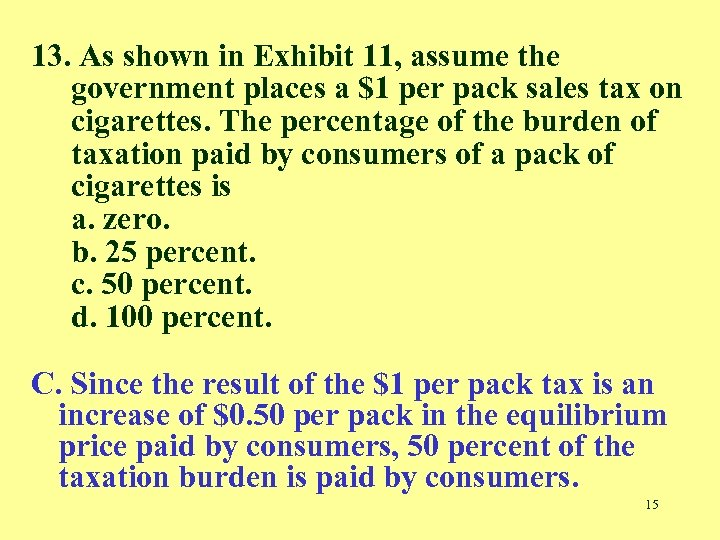13. As shown in Exhibit 11, assume the government places a \$1 per pack sales tax on cigarettes. The percentage of the burden of taxation paid by consumers of a pack of cigarettes is a. zero. b. 25 percent. c. 50 percent. d. 100 percent. C. Since the result of the \$1 per pack tax is an increase of \$0. 50 per pack in the equilibrium price paid by consumers, 50 percent of the taxation burden is paid by consumers. 15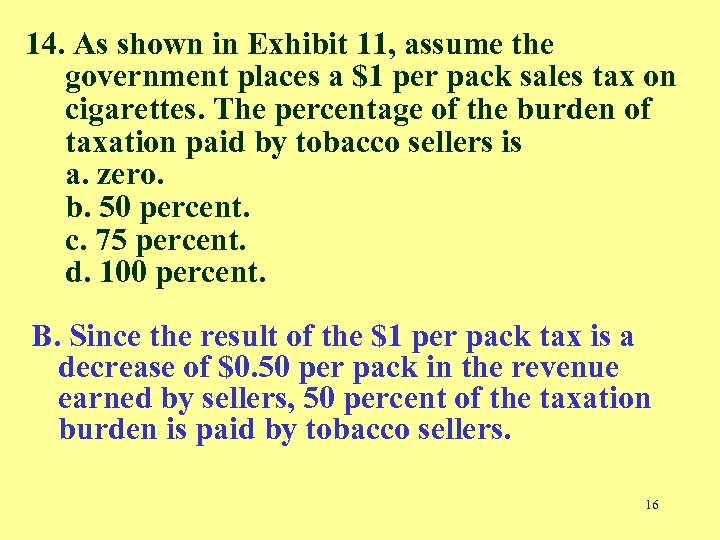14. As shown in Exhibit 11, assume the government places a \$1 per pack sales tax on cigarettes. The percentage of the burden of taxation paid by tobacco sellers is a. zero. b. 50 percent. c. 75 percent. d. 100 percent. B. Since the result of the \$1 per pack tax is a decrease of \$0. 50 per pack in the revenue earned by sellers, 50 percent of the taxation burden is paid by tobacco sellers. 16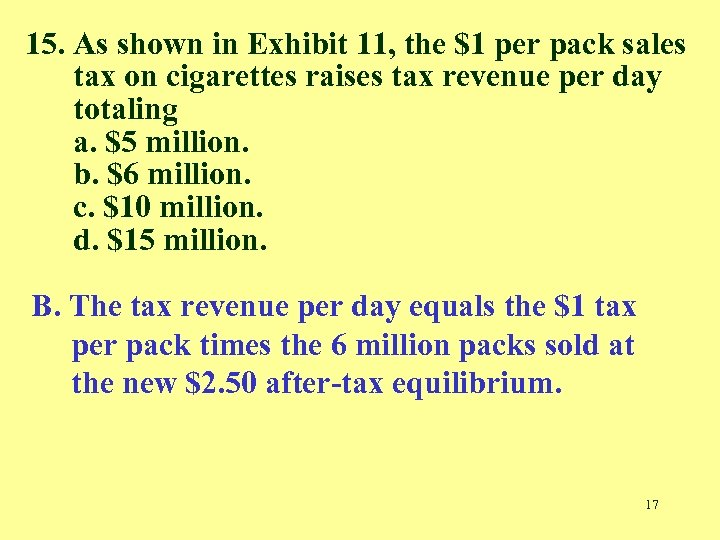15. As shown in Exhibit 11, the \$1 per pack sales tax on cigarettes raises tax revenue per day totaling a. \$5 million. b. \$6 million. c. \$10 million. d. \$15 million. B. The tax revenue per day equals the \$1 tax per pack times the 6 million packs sold at the new \$2. 50 after-tax equilibrium. 17